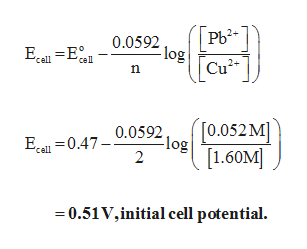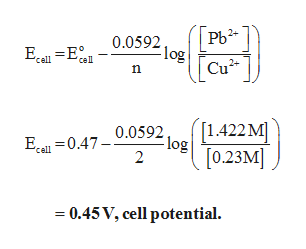# A voltaic cell consists of a Pb/Pb2+ half-cell and a Cu/Cu2+ half-cell at 25 ∘C. The initial concentrations of Pb2+ and Cu2+ are 0.0520 M and 1.60 M , respectively. a) What is the initial cell potential? Express your answer using two significant figures.b) What is the cell potential when the concentration of Cu2+ has fallen to 0.230 M ? Express your answer using two significant figures.c) What is the concentration of Pb2+ when the cell potential falls to 0.370 V ? Express your answer using three significant figures.

Question
49 views

A voltaic cell consists of a Pb/Pb2+ half-cell and a Cu/Cu2+ half-cell at 25 ∘C. The initial concentrations of Pb2+ and Cu2+ are 0.0520 M and 1.60 M , respectively.

a) What is the initial cell potential? Express your answer using two significant figures.

b) What is the cell potential when the concentration of Cu2+ has fallen to 0.230 M ? Express your answer using two significant figures.

c) What is the concentration of Pb2+ when the cell potential falls to 0.370 V ? Express your answer using three significant figures.

check_circle

Step 1

a)

Reaction at cathode:

Cu2+ + 2e- --> Cu(s)     Eocathode = 0.34 V

Reaction at anode:

Pb (s) --> Pb2+ + 2e-   Eoanode = -0.13 V

Number of moles of electrons involved, n = 2

Standard cell potential, Eo = Eocathode - Eoanode = 0.34 V- (-0.13 V) = 0.47 V.

Initial cell potential can be calculated as follows,help_outlineImage TranscriptioncloseР- 0.0592 -log cell Го.052M) [1.60м] 0.0592 -log 2 Ecl0.47- cell =0.51V,initial cell potential fullscreen
Step 2

b)

The cell reaction is given below.

Cu2+ + Pb (s)  --> Cu(s) + Pb2+

The new concentration of Cu2 = 0.23 M

Change in concentration of Cu2 = 1.6 M – 0.23 M = 1.37 M

Theref...help_outlineImage TranscriptionclosePb2 Flog Cu2 0.0592 cell n 1422M -log [0.23M 0.0592 Ecl0.47- cell 2 0.45 V, cell potential fullscreen

### Want to see the full answer?

See Solution

#### Want to see this answer and more?

Solutions are written by subject experts who are available 24/7. Questions are typically answered within 1 hour.*

See Solution
*Response times may vary by subject and question.
Tagged in

### Electrochemistry